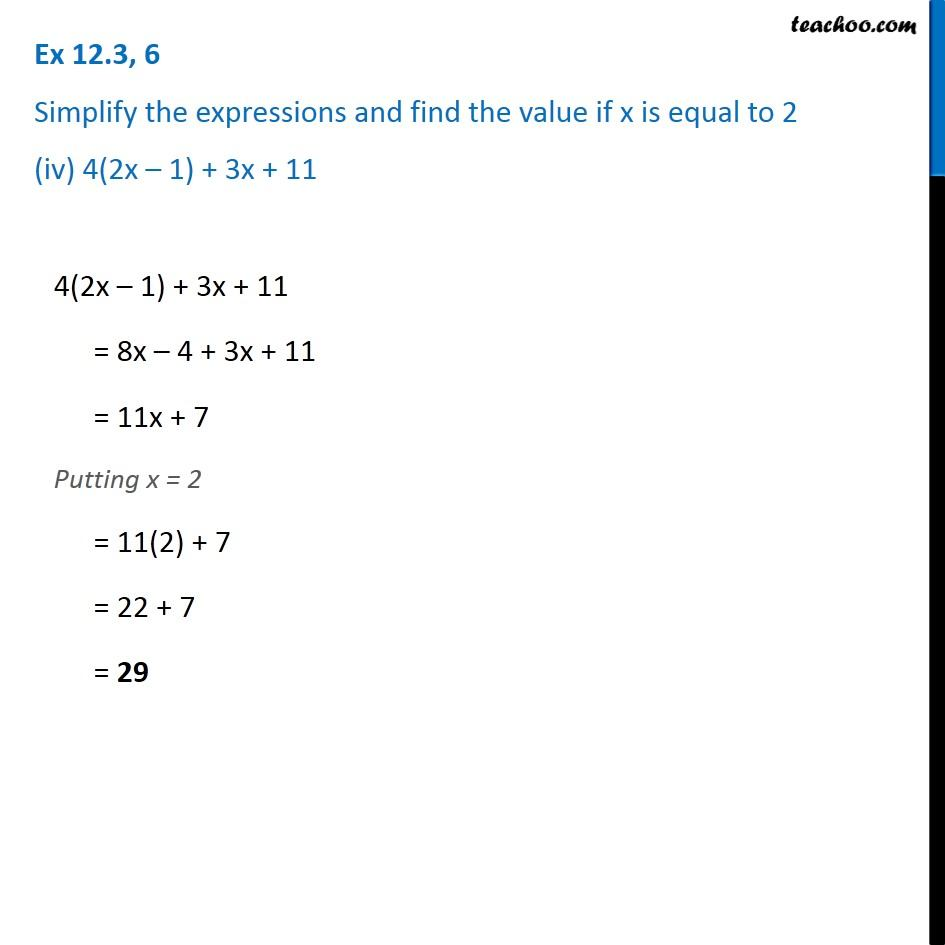Ex 12.3

Chapter 12 Class 7 Algebraic Expressions
Serial order wiseIntroducing your new favourite teacher - Teachoo Black, at only ₹83 per month

### Transcript

Ex 12.3, 6 Simplify the expressions and find the value if x is equal to 2 (iv) 4(2x – 1) + 3x + 11 4(2x – 1) + 3x + 11 = 8x – 4 + 3x + 11 = 11x + 7 Putting x = 2 = 11(2) + 7 = 22 + 7 = 29